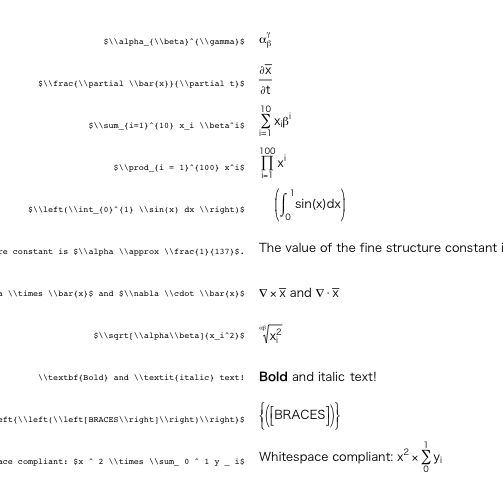# latex2exp: Use LaTeX Expressions in Plots

LaTeX風の表記をRのプロットに取り込む> library(latex2exp)


バージョン: 0.3.3

latex2exp Converts a LaTeX string to a 'plotmath' expression.
latex2exp_examples Plots a number of example LaTeX string, as parsed by 'latex2exp'.
latex2exp_supported Returns a list of all supported LaTeX symbols and expressions that can be converted with 'latex2exp'.
plot.expression Plots an expression on the current graphical device.
print.latextoken Prints out a parsed LaTeX object, as returned by latex2exp(..., output='ast').
toString.latextoken Converts a token created by latex2exp to a string, later to be parsed into an expression (for internal use).

## latex2exp

### Arguments

• string
• output
> latex2exp("$\\alpha$")

expression($\alpha$ = paste("", "", alpha, , , , ""))

> latex2exp("The ratio of 1 and 2 is $\\frac{1}{2}$")

expression(The ratio of 1 and 2 is $\frac{1}{2}$ = paste("The ratio of 1 and 2 is ",
"", frac(paste("1"), paste("", "2")), ""))


## latex2exp_examples()

> latex2exp_examples()## latex2exp_supported

> latex2exp_supported(plot = FALSE)

  "\\div"         "\\pm"          "\\neq"         "\\geq"
 "\\leq"         "\\approx"      "\\sim"         "\\propto"
 "\\equiv"       "\\cong"        "\\in"          "\\notin"
 "\\cdot"        "\\times"       "\\subset"      "\\subseteq"
 "\\nsubset"     "\\supset"      "\\supseteq"    "\\rightarrow"
 "\\leftarrow"   "\\Rightarrow"  "\\Leftarrow"   "\\forall"
 "\\exists"      "\\%"           "\\ast"         "\\perp"
 "\\bullet"      "\\Im"          "\\Re"          "\\wp"
 "\\otimes"      "\\oplus"       "\\oslash"      "\\surd"
 "\\neg"         "\\vee"         "\\wedge"       "\\sqrt"
 "\\sum"         "\\prod"        "\\int"         "\\frac"
 "\\bigcup"      "\\bigcap"      "\\lim"         "\\overset"
 "\\normalsize"  "\\small"       "\\tiny"        "\\textbf"
 "\\textit"      "\\mathbf"      "\\mathit"      "\\mathrm"
 "\\infty"       "\\partial"     "\\cdots"       "\\ldots"
 "\\degree"      "\\clubsuit"    "\\diamondsuit" "\\heartsuit"